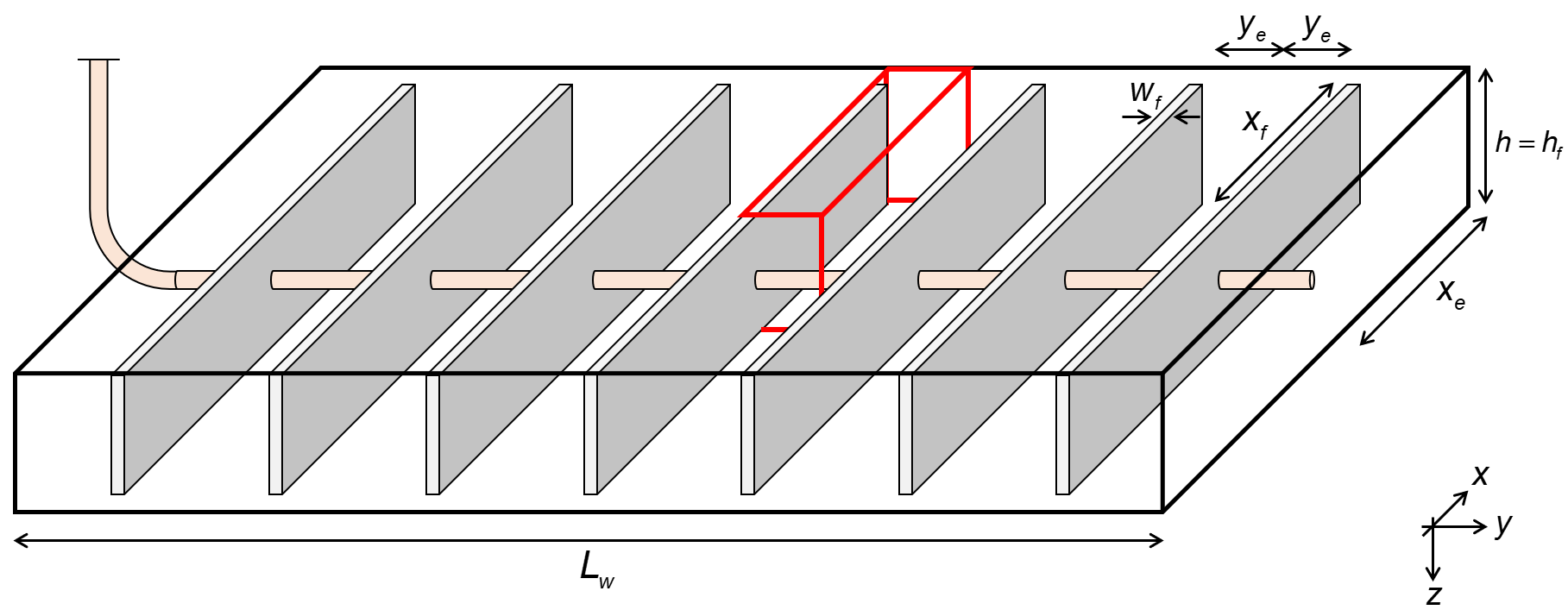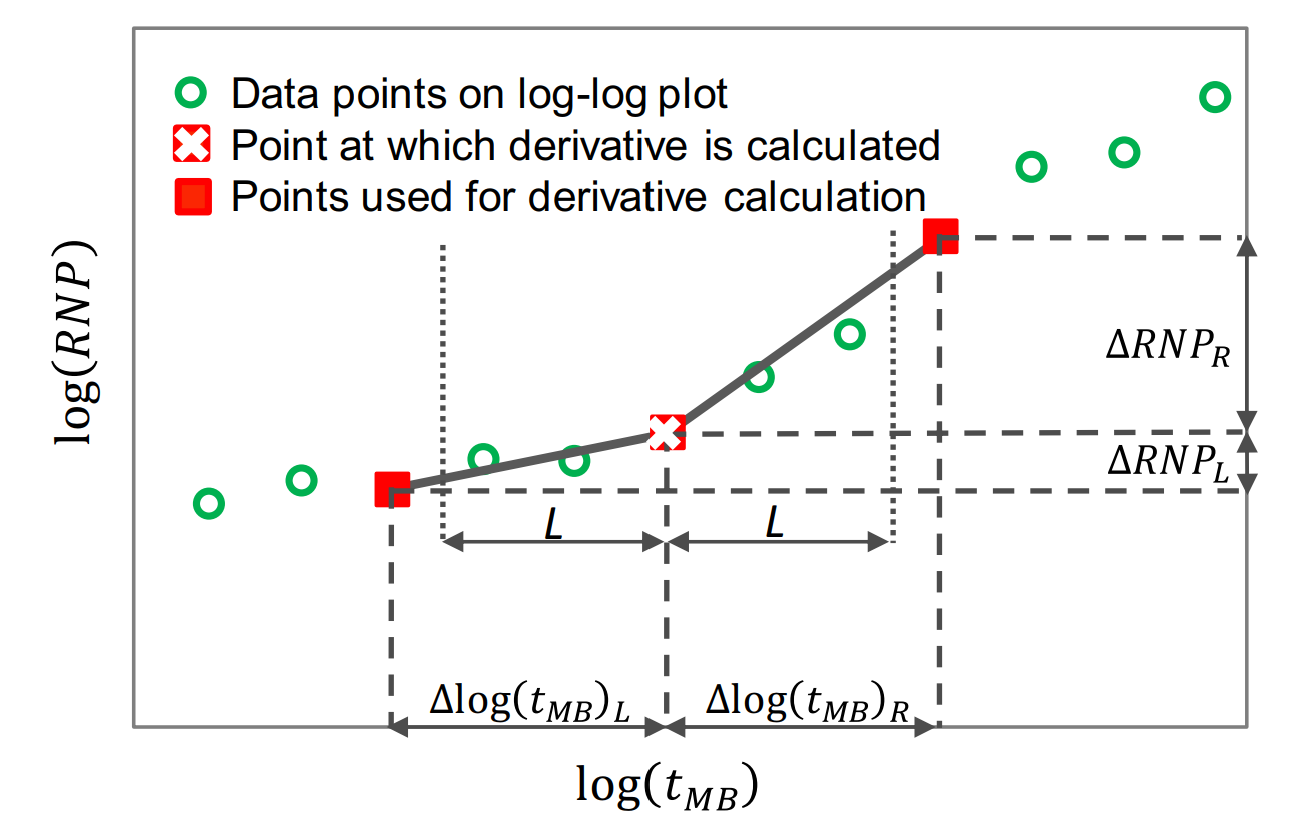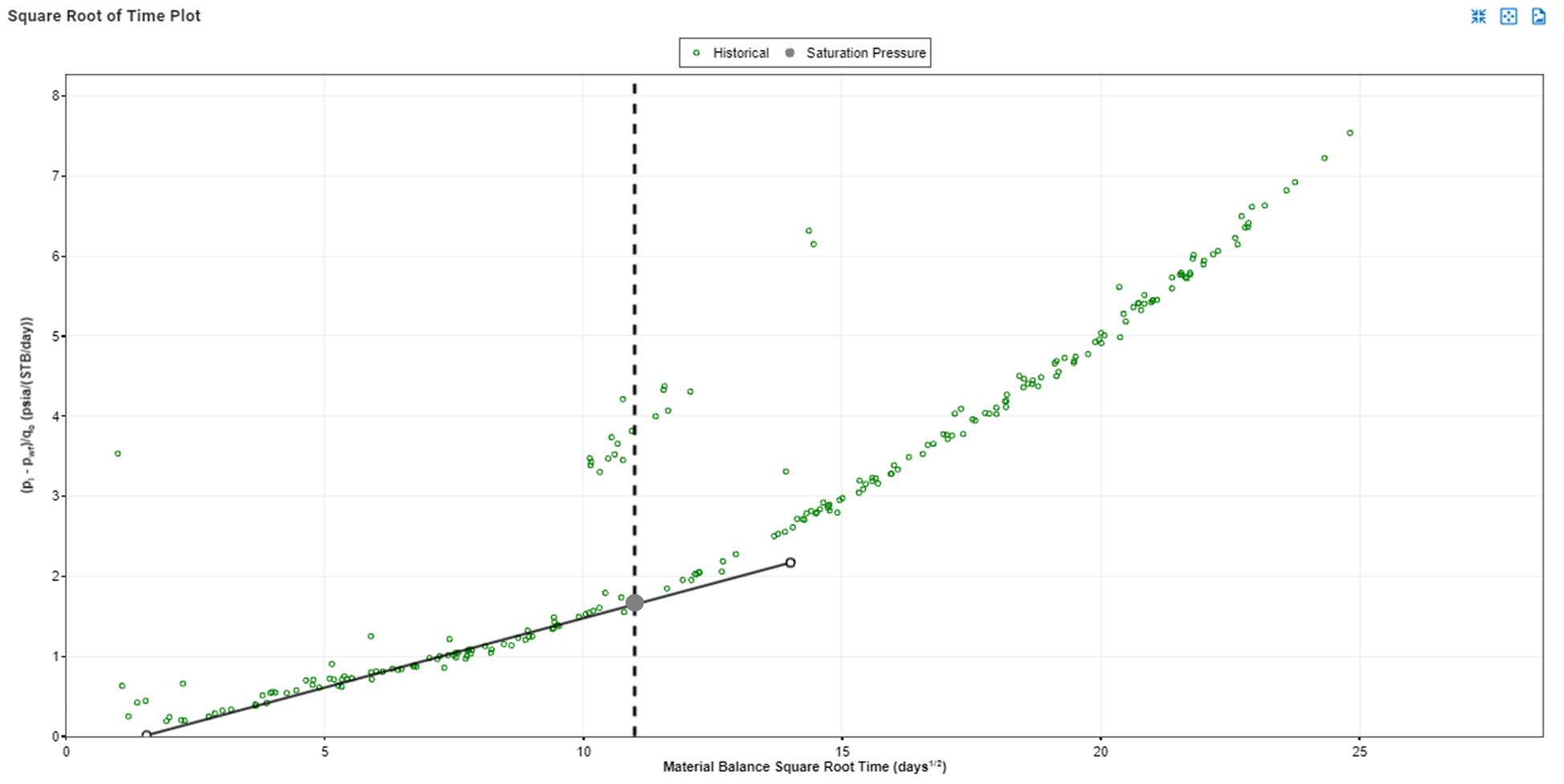# Classical RTA

## 1. Theory

There have been many studies showing that infinite acting, linear flow is the dominant flow regime in multifractured horizontal wells for tight unconventional reservoirs. Most common production analysis of linear flow was first introduced by Wattenbarger et al. in 1998. They proposed analytical solutions for both constant rate and constant pressure. In reality, rates and pressures are changing simultaneously. To account for that, the principle of superposition is applied.

In 1993, Palacio and Blasingame (1993) introduced the concept of material balance time (MBT). Material balance time

• is a superposition time function.
• converts variable rate data into an equivalent constant rate solution.
• is rigorous for a boundary dominated flow regime
• works well for transient data also, but is only an approximation (errors can be up to 20% for linear flow)

In the whitson+ classical RTA module, material balance time is the default time function, hence the theory for the constant rate solution is emphasized in this part of the manual. On the other hand, there is an option to use constant pressure solution by selecting "Real Time" as a function of time as presented here.

The purpose of conducting this analysis is to determine the linear flow parameters (LFP=$$A\sqrt{k}$$) and drainage volume by interpreting production data. By using Arps equation with respect to time to end of linear flow $$(t_{elf})$$, production forecast can be made.

### 1.1 AssumptionsFig. 1: Wellbox model

Fig.1 shows the geometry of the well used as a base assumption of these analyses. The fracture is assumed to have infinite fracture conductivity, such that the linear flow is perpendicular to the fracture. Additionally, this module assumes single-phase flow with no flow beyond the fracture tip ($$x_e=x_f$$ and $$h=h_f$$), i.e. a fully-penetrating fracture (1D-model).

### 1.2 Superposition Time

There are two superposition time function options in whitson+; material balance time and linear superposition time. In addition to these, one can use real time (called "Variable Pressure" in other tools), in which no superposition time function has been applied.

#### 1.2.1 Material Balance Time - DEFAULT

The use of material balance time is common in the rate transient analysis, and is the default in whitson+. Material balance time

• is a superposition time function.
• converts variable rate data into an equivalent constant rate solution.
• is rigorous for a boundary dominated flow regime
• works well for transient data also, but is only an approximation (errors can be up to 20% for linear flow)

Mathematically, material balance time, MBT $$(t_c)$$ is expressed in:

where $$Q(t)$$ and $$q(t)$$ are cumulative production and production rate at time $$t$$.

#### 1.2.2 Linear Superposition Time

Mathematically, linear superposition time, $$(t_{\text{linear}})$$ is expressed as:

### 1.3 Phase

You can select to run the classical RTA analysis with either of the following rates

• Oil (Surface)
• Gas (Surface)
• Hydrocarbon (Reservoir)
• Liquid (Reservoir)
• Total (Reservoir)

Hydrocarbon reservoir rates are calculated from surface rates using PVT and bottomhole pressures as follows

Liquid reservoir rates are calculated from surface rates using PVT and bottomhole pressures as follows

Total reservoir rates are calculated from surface rates using PVT and bottomhole pressures as follows

In which $$q_{g,res}$$, $$q_{g,res}$$ and $$q_{w,res}$$ can be calculated as follows

The PVT properties solution GOR, $$R_{s}$$, oil formation volume factor, $$B_{o}$$, solution CGR, $$r_{s}$$, gas formation volume factor, $$B_{gd}$$, and water formation volume factor, $$B_{w}$$ is all evaluated at the flowing bottomhole pressure, $$p_{wf}$$, every given day to calculate total rates.

### 1.4 Rate Normalized Pressure (RNP)

Rate Normalized Pressure (RNP) is very useful for production analysis where flowing pressures and rates change through time. It is defined as the flowing pressure drop divided by rate.

### 1.5 Pressure Normalized Rate (PNR)

Pressure normalized rate is the inverse of rate normalized pressure. Pressure normalized rate is very useful for production analysis where flowing pressures and rates change through time. It is defined as the rate divided by flowing pressure drop.

### 1.6 RTA Derivatives

Derivatives assists with flow regime identification and can be calculated for both pressure (p), rate normalized pressure (RNP) and pressure normalized rate (PNR).

Derivative analysis amplifies the reservoir signal, but also amplfies the noise. - Dave Anderson

#### 1.6.1 Derivative Options

The logarithmic derivative used in RTA applied directly on the rate normalized pressure (RNP) data is given by, i.e., .

There are three different ways to compute this derivative in whitson+:

• The Bourdet Derivative (Bourdet et al., 1989)
• Weighted Central Difference
• Central Difference

The Bourdet derivative is commonly used in well-test analysis (PTA) for identifying flow regimes (Lee et al., 2003) and has similarly found utility in RTA.

Governing Equation

To calculate the Bourdet derivative (Bourdet et al., 1989) at any given point, one point before (left) and one point after (right) is used.

Smoothing

The Bourdet approach is illustrated in Fig. 2 and is a way to calculate and smooth the derivative based on a log-cycle window size, L.Fig. 2: Illustration of a derivative calculating using the Bourdet method. Image courtesy of Samaneh Moghadam (April, 2020). Modified from Lee et al. (2003).

It is different from a weighted central difference in the way that it uses a constant log-cycle window size, L, on both sides of the point of derivation. A weighted central difference, on the other hand, uses a constant index step size, e.g. i-5 and i+5 for step size of 5, on each side of the point of derivation. The window size, L, represents the log-cycle fraction used to control the amount of smoothing. Typical values are 0.01 to 0.2. The default L in whitson+ is 0.2. The larger the L value, the more smoothing.

The Bourdet algorithm implemented in whitson+ was provided by Behnam Zanganeh.

#### 1.6.3 Weighted Central Difference

The weighted central difference is given by,

where the $$L$$-subscript refers to the index to the left with a constant step size, $$S$$, i.e. $$i-S$$ and the $$R$$-subscript refers to the index to the right with a constant step size, $$S$$, i.e. $$i+S$$.

#### 1.6.4 Central Difference

This is the naive implementation, given by three points per derivative for central difference in the following manner

#### 1.6.5 Derivatives in Material Balance Time

Because of noise in real field data, material balance time may not be in sequence. This might cause problems in derivative calculations. Hence, the data must be sorted in terms of increasing material balance time prior to calculating the derivative. The following procedure, adapted from Samandarli et al.(2012), is used for this purpose.

1. RNP and material balance time $$(t_c)$$ are calculated at each real time step.
2. RNP and $$(t_c)$$ are sorted in increasing order of material balance time, with repeat material balance times eliminated.
3. The RNP derivative, RNP', is then calculated using the sorted data.

### 1.7 RTA Integrals

In the case of complex and noisy data, Blasingame et al.  introduced the concepts of pressure-integral and pressure-integral difference which have become essential tools in scrutiny of well-test data throught type-curve analysis. Similarly, McCray (1990) presented the idea of the rate-integral and rate-integral derivative which was utilized by Blasingame and his students and colleagues for type-curve analysis of production data in various publications.

### 1.7.1 The Rate Normalized Pressure (RNP) Integral

The use of integral of the RNP $$\left(IRNP\right)$$ and its derivative $$\left(IRNP'_R\right)$$ was suggested by Chu et al.  for the study of flow-regime signature in the Wolfcamp shale. For liquids, the equations are written as follows:

The integral can be calculated using various time functions (real time, linear superposition time). However, it is presented here in material balance time for example purposes.

As with $$\left(RNP\right)$$ and $$\left(RNP'_R\right)$$ the calculations for $$\left(IRNP\right)$$ and $$\left(IRNP'_R\right)$$ can be applied to gases through inclusion of gas pseudovariables.

### 1.7.2 Discrete Calculation

The discrete form of Eq. \eqref{eq:RTA-integral} is expressed in the following manner:

The conversion from a slope to LFP (A√k) while using the RNP integral must be adjusted by 2/3 to ensure that the LFP is calculated correctly from the slope. This follows from integrating the RNP as a function of √t.

When turning on the integral function, this correction is applied

### 1.8 Solutions of Linear Flow - Surface Oil Rates

Combining constant rate (variable pressure) solution with superposition time allows to account for variable rate solution.

Theoretically, linear flow can be defined in terms of square root of time as follows

For oil:

For gas:

For water:

For hydrocarbon:

For liquid:

For total:

Note

For liquid rates, a "pseudo" liquid viscosity is calculated as the saturation weighted average viscosity, as follows

For total rates, a "pseudo" total viscosity is calculated as the saturation weighted average viscosity, as follows

in which $$B_o$$ is oil formation volume factor in RB/STB, $$B_w$$ is water formation volume factor in RB/STB, $$T_R$$ is reservoir temperature in rankine, $$\phi$$ is porosity in fraction, $$\mu$$ is viscosity in cp, $$c_t$$ is total compressibility in 1/psia and $$k$$ is permeability in md. Fracture height and half-length in feet are denoted by $$h$$ and $$x_f$$, respectively. Initial and flowing bottomhole pressure are $$p_i$$ and $$p_{wf}$$ in psia. Water viscosity, $$\mu_w$$, is set to 0.5 cp.

Total compressibility accounts for the compressibility of the fluid phases present in the system as well as the rock compressibility and is written as

where $$c_g$$, $$c_o$$, $$c_w$$ and $$c_r$$ denote gas, oil, water and formation (rock) compressibility, respectively. The default value of $$c_r$$ is 4x10$$^{-6}$$ 1/psia and $$c_w$$ is 2.8x10$$^{-6}$$ 1/psia in whitson+.

$$\Delta m(p)$$ is real gas pseudo-pressure drop defined as

According to Eqs. \eqref{eq:CR-linear-oil}, \eqref{eq:CR-linear-gas}, \eqref{eq:CR-linear-water} the plot of rate normalized pressure $$\left(\frac{\Delta p}{q}\right)$$ vs. square root of time $$\sqrt{t}$$ (or square root of material balance time $$\sqrt{t_c})$$ generates a straight line. The slope of this line is given by

For oil:

For gas:

For water:

For hydrocarbon, liquid and total:

Subscript $$CR$$ is to emphasize the variable of constant rate solution.

### 1.9 Skin and Fracture Conductivity

The intercept ($$b'$$) from the linear flow equation is a result of near well effects, e.g. skin $$(s')$$ and finite fracture conductivity $$(F'_{cd})$$. The unit of the intercept is psia/STB/d.

Skin and fracture conductivity are defined as follows:

For oil:

For gas:

For water:

For hydrocarbon, liquid and total:

Where $$r_w$$ (equal 1 ft) indicates well radius in ft and $$n_f$$ represents effective fractures number.

### 1.10 Linear Flow Parameter

The linear flow parameter (LFP) is expressed in terms of slope of the straight line as

For oil:

For gas:

For water:

For hydrocarbon, liquid and total:

The unit of LFP is ft$$^2$$md$$^{\frac{1}{2}}$$.

### 1.11 Distance of Investigation

It is important to be able to establish the end of the linear flow where the slope on the square root of time plot start to depart from being a straight line. The time to end of linear flow $$(t_{elf})$$ is key to specify the drainage volume, and to resolve estimates of parameters such as $$k$$ and $$x_f$$.

Generally, distance of investigation $$(y_e)$$ can be determined by dividing well horizontal length $$(L_w)$$ with effective fractures number $$(n_f)$$.

The constant rate solution of $$y_e$$, or the distance to the outer boundary is decribed as

Combining Eqs. \eqref{eq:ye-Lw} and \eqref{eq:CR-ye} gives

The time to end of linear flow $$(t_{elf})$$ can be solved by rearranging Eq. \eqref{eq:CR-ye-2}

In the case of no deviation of production data from straight line, the latest production time should be used as $$t_{elf}$$ which will generate the minimum drainage volume.

### 1.12 Permeability and Fracture Half-length

#### 1.12.1 Permeability

Rearranging Eq. \eqref{eq:CR-ye-2} to get permeability will result in

#### 1.12.2 Fracture half-length

LFP can also be defined as

in which $$A$$ is total effective fracture surface area in ft$$^2$$ defined as

After determining LFP and $$k$$ previously, $$x_f$$ can be calculated straightforward by rearranging Eqs. \eqref{eq:LFP} and \eqref{eq:total-area-to-flow}.

### 1.13 Contacted Pore Volume, Drainage Area and Hydrocarbons-in-place

Having all the necessary variables, the contacted pore volume in ft$$^3$$ can be easily determined by:

Drainage area $$A_d$$ in acres (1 acre = 43,560 ft$$^2$$) is defined as

An alternative way to calculate drainage $$A_d$$ is

In which 7758 is a conversion factor from acre-ft to bbl.

Original hydrocarbons-in-place are calculated using the following relationships:

For oil:

For gas:

For water:

### 1.14 Production Forecast

With simple decline curve analysis, the production forecast can be projected. The classic arps hyperbolic decline is written as

Flow production rate and nominal decline rate at the start of forecast are depicted by $$q_i$$ and $$a_i$$, respectively. Flow production rate at time $$t$$ is $$q(t)$$ while $$b$$ is rate exponent. In this module, the production forecast is start at the end of the linear flow $$(t_{elf})$$, thus the other variables are with respect to $$t_{elf}$$.

Production forecast rate is calculated by rewritting Eq. \eqref{eq:arps-dca}

A more detailed explanation of decline curve analysis can be found here.

### 1.15 Geomechanical Effects - Pressure-Dependent Permeability

For gas reservoirs, the matrix can be assigned a pressure-dependent permeability on the form

where $$k_m(p)$$ is a dimensionless permeability multiplier.

and $$\gamma_r$$ is a property that be assigned in the frontend. To activate the pressure-dependent permeability, make $$\gamma_r$$ a non-zero value.

To incorporate a variation of permeability with pressure into the pseudo-pressure term, the definition of pseudo-pressure is modified as follows

Geomechanical effects can also be added in both the matrix and fracture in the numerical model.

### 1.16 Computing Original Gas in Place with Adsorption

Ambrose et al. argue that the free OGIP must be corrected for the adsorbed gas that occupies some of the hydrocarbon pore volume (HCPV). If porosity is estimated from core plugs where core preparation has removed the adsorbed gas, then the OGIP will be overestimated.

The convention of reporting OGIP when adsorption is included, is to report it in units of scf/ton (standard cubic feet per short ton). For a fluid system having only dry/wet gas and water, the total OGIP is

where

and with $$C_1\approx 5.7060$$ and $$C_2\approx1.318\cdot10^{-6}$$ are unit conversion factors.

The OGIP resolved in GFMB is the free OGIP. We can use this volume to compute the rock mass, which in turn can be used to calculate the adsorbed OGIP. Multiplying equation \eqref{eq:freegasperton} with the rock mass ($$G=\hat{G}_f m_r$$) gives the free OGIP in units of scf. Solving for the rock mass yields

This rock mass can then be multiplied by equation \eqref{eq:adsorbedgasperton} to get the adsorbed OGIP in scf, i.e.,

#### Total Compressibility when Adsorption is ON

In order to honor mass balance, it becomes necessary to modify total system compressibility when accounting for adsorption. Total system compressibility can be determined using the following equation:

The derivation of this equation is shown here and is contructed upon Ambrose et al..

### 1.17 Limitations of Classical RTA

One of the key limitations of classical RTA is the single-phase flow assumption. This is a good assumption for dry and wet gas reservoirs, and when producing with flowing pressures above the saturation pressure. However, for wells producing below the saturation pressure, the single-phase flow assumption is not valid. Hence, using the classical RTA equations for wells subject to multiphase flow effects might skew the interpretation. If the saturation pressure coincides with the change of slope on the square root of time plot, as seen in Fig.2, the slope change might just be an artifact of multiphase flow. To account for the multiphase flow effects, we recommend using the numerical RTA workflow. More info can be found here.Fig. 2: Slope change coinciding with well dropping below the saturation pressure might be an artifact of multiphase flow.

## 2. Comments on Use of Material Balance Time

• If the analytical solutions are plotted to fully-penetrating fracture problem for either a constant rate or constant pressure case (Eqs. 2 and 3 in Wattenbarger et al. (1998)), a half-slope on a log-log plot should be expected during infinite acting (IA) period with a constant difference between the two solutions of a factor $$\frac{\pi}{2}$$. The constant pressure solution in the boundary-dominated flow (BDF) period will exhibit an exponential function, whereas the constant rate solution in the BDF will exhibit a unit-slope.

• If one plots the constant pressure solution versus material balance time, then the curve will (1) overlap the constant rate solution in the BDF period (because material balance time applies rigorously in the BDF period) and, (2) approximate the constant rate solution in the IA period (i.e. it plots close to the true solution, but not on top of it as in the BDF period).

• Because the material balance time solution is only an approximate solution in the IA period, the resulting key numbers (e.g. $$x_f$$ and $$k$$) will not be the exact numbers you expect, at least for simple synthetic cases (e.g. a low GOR oil with constant $$p_{wf}$$). However, for a variable rate and pressure solution (real life), one can only get the true values by rigorous superposition (or de-superposition), and thus an approximate solution by the use of material balance time is likely good enough.

## 3. Constant Flowing Pressure Solution

The constant flowing pressure solution is the opposite of constant rate solution. Instead of having constant rate, it employs variable rate while $$p_{wf}$$ is assumed constant.

The workflow of classical RTA is the same for both solutions, the only difference is the equations being used in the calculations. It is worth to mention that there is a factor of $$\pi/2$$ difference between the constant rate and constant pressure solutions.

The following equations are applied for contant pressure solution:

For oil:

For gas:

For water:

For hydrocarbon:

For liquid:

For total:

#### Slope of straight line

For oil:

For gas:

For water:

For hydrocarbon, liquid and total:

#### Linear Flow Parameter

For oil:

For gas:

For water:

For hydrocarbon, liquid and total: